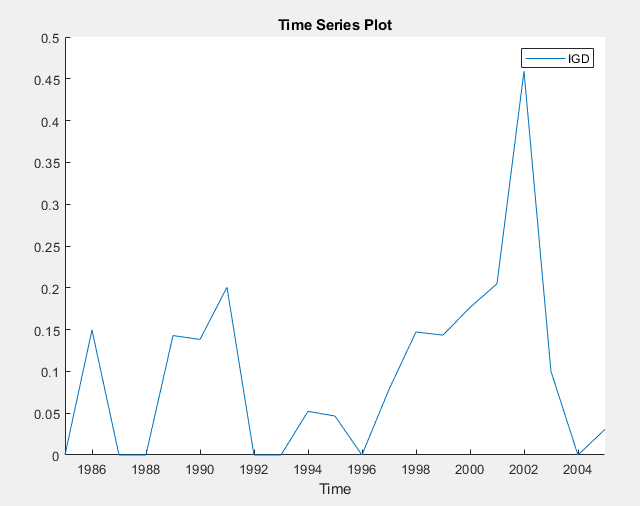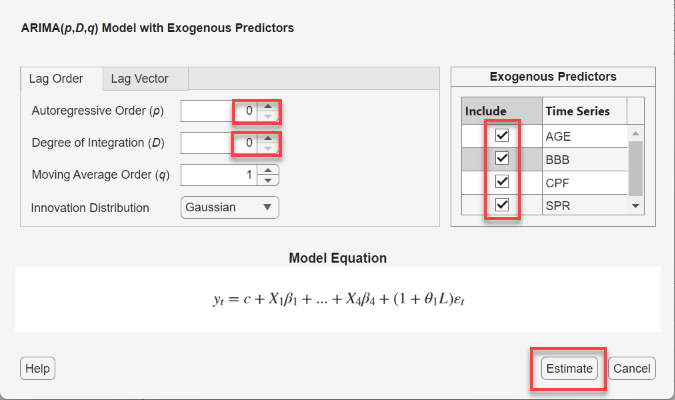## Estimate ARIMAX Model Using Econometric Modeler App

This example shows how to specify and estimate an ARIMAX model using the Econometric Modeler app. The data set, which is stored in `Data_CreditDefaults.mat`, contains annual investment-grade corporate bond default rates, among other predictors, from 1984 through 2004. Consider modeling corporate bond default rates as a linear, dynamic function of the other time series in the data set.

### Import Data into Econometric Modeler

At the command line, load the `Data_CreditDefaults.mat` data set.

`load Data_CreditDefaults`

For more details on the data set, enter `Description` at the command line.

Convert the table `DataTable` to a timetable:

1. Clear the row names of `DataTable`.

2. Convert the sampling years to a `datetime` vector.

3. Convert the table to a timetable by associating the rows with the sampling times in `dates`.

```DataTable.Properties.RowNames = {}; dates = datetime(dates,12,31,'Format','yyyy'); DataTable = table2timetable(DataTable,'RowTimes',dates);```

At the command line, open the Econometric Modeler app.

`econometricModeler`

Alternatively, open the app from the apps gallery (see Econometric Modeler).

Import `DataTable` into the app:

1. On the Econometric Modeler tab, in the Import section, click.

2. In the Import Data dialog box, in the Import? column, select the check box for the `DataTable` variable.

3. Click .

The variables, including `IGD`, appear in the Data Browser, and a time series plot containing all the series appears in the Time Series Plot(AGE) figure window.

### Assess Stationarity of Dependent Variable

In the Data Browser, double-click `IGD`. A time series plot for `IGD` appears in the Time Series Plot(IGD) figure window.`IGD` appears to be stationary.

Assess whether `IGD` has a unit root by conducting a Phillips-Perron test:

1. On the Econometric Modeler tab, in the Tests section, click > Phillips-Perron Test.

2. On the PP tab, in the Parameters section, set Number of Lags to `1`.

3. In the Tests section, click .

The test results in the Results table of the PP(IGD) document.The test rejects the null hypothesis that `IGD` contains a unit root.

### Inspect Correlation and Collinearity Among Variables

Plot the pairwise correlations between variables.

1. Select all variables in the Data Browser by clicking `AGE`, then press Shift and click `SPR`.

2. Click the Plots tab, then click Correlations.

A correlations plot appears in the Correlations(AGE) figure window.All predictors appear weakly associated with `IGD`. You can test whether the correlation coefficients are significant by using `corrplot` at the command line.

Assess whether any variables are collinear by performing Belsley collinearity diagnostics:

1. In the Data Browser, select all variables.

2. Click the Econometric Modeler tab. Then, in the Tests section, click > Belsley Collinearity Diagnostics.

Tabular results appear in the Collinearity(AGE) document.None of the condition indices are greater than the condition-index tolerance (`30`). Therefore, the variables do not exhibit multicollinearity.

### Specify and Estimate ARIMAX Model

Consider an ARIMAX(0,0,1) model for `IGD` containing all predictors. Specify and estimate the model.

1. In the Data Browser, click `IGD`.

2. Click the Econometric Modeler tab. Then, in the Models section, click the arrow to display the models gallery.

3. In the models gallery, in the ARMA/ARIMA Models section, click .

4. In the ARIMAX Model Parameters dialog box, on the Lag Order tab, set Moving Average Order to `1`.

5. In the Predictors section, select the Include? check box for each time series.6. Click . The model variable `ARIMAX_IGD` appears in the Models section of the Data Browser, and its estimation summary appears in the Model Summary(ARIMAX_IGD) document.At a 0.10 significance level, all predictors and the MA coefficient are significant.

Close all figure windows and documents.

### Check Goodness of Fit

Check that the residuals are normally distributed and uncorrelated by plotting a histogram, quantile-quantile plot, and ACF of the residuals.

1. In the Data Browser, select `ARIMAX_IGD`.

2. On the Econometric Modeler tab, in the Diagnostics section, click > Residual Histogram.

3. Click > Residual Q-Q Plot.

4. Click > Autocorrelation Function.

5. In the right pane, drag the Histogram(ARIMAX_IGD) and QQPlot(ARIMAX_IGD) figure windows so that they occupy the upper two quadrants, and drag the ACF so that it occupies the lower two quadrants.The residual histogram and quantile-quantile plots suggest that the residuals might not be normally distributed. According to the ACF plot, the residuals do not exhibit serial correlation. Standard inferences rely on the normality of the residuals. To remedy nonnormality, you can try transforming the response, then estimating the model using the transformed response.